# CLASS 12 PHYSICS CHAPTER 1-ELECTRIC CHARGES AND FIELDS

Question1. What is the force between two small charged spheres having charges of 2 × 10−7 C and 3 × 10−7 C placed 30 cm apart in air?

Solution :

The formula used to find the force, F is given as,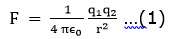Where, q1 and q2 are the charges.
r is the distance between the charges.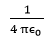is a constant and its value is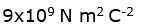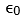is the permittivity of free space. Its value is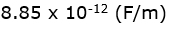Now, the diagram is: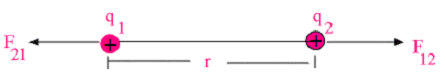Since, both the charges are positive, thus, the nature of force will be repulsive. F12 is the force on charge q1 caused by charge q2.
Now, Given:
Charge on the first sphere,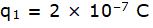Charge on the second sphere,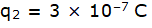Distance between the spheres, r = 30 cm = 0.3 m
Putting the values in equation (1), we get,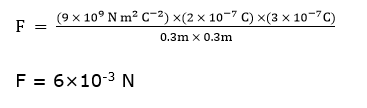Hence, the force between the given charged particles will be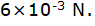Since the nature of the charges is the same i.e. they are both positive. Hence, the force will be repulsive.

Question2. The electrostatic force on a small sphere of charge 0.4 μC due to another small sphere of charge − 0.8 μC in air is 0.2 N. (a) What is the distance between the two spheres? (b) What is the force on the second sphere due to the first?

Solution :
(a) Electrostatic force on the first sphere, F = 0.2 N

Charge on this sphere, q1 = 0.4 μC = 0.4 × 10−6 C

Charge on the second sphere, q= − 0.8 μC = − 0.8 × 10−6 C

Electrostatic force between the spheres is given by the relation,

Where, ∈0 = Permittivity of free space

The distance between the two spheres is 0.12 m.

(b) Both the spheres attract each other with the same force. Therefore, the force on the second sphere due to the first is 0.2 N.

Question3. Check that the ratio ke2/G memp is dimensionless. Look up a Table of Physical Constants and determine the value of this ratio. What does the ratio signify?

Solution :
The given ratio is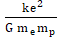.

Where,

G = Gravitational constant

Its unit is N mkg−2.

me and mp = Masses of electron and proton.

Their unit is kg.

e = Electric charge.

Its unit is C.

0 = Permittivity of free space

Its unit is N mC−2.

Hence, the given ratio is dimensionless.

e = 1.6 × 10−19 C

G = 6.67 × 10−11 N mkg-2

me= 9.1 × 10−31 kg

mp = 1.66 × 10−27 kg

Hence, the numerical value of the given ratio is

This is the ratio of electric force to the gravitational force between a proton and an electron, keeping distance between them constant.

Question4. (a) Explain the meaning of the statement ‘electric charge of a body is quantised’.

(b) Why can one ignore quantisation of electric charge when dealing with macroscopic i.e., large scale charges?

Solution :
(a) Electric charge of a body is quantized. This means that only integral (1, 2, …., n) number of electrons can be transferred from one body to the other. Charges are not transferred in fraction. Hence, a body possesses total charge only in integral multiples of electric charge.

(b) In macroscopic or large scale charges, the charges used are huge as compared to the magnitude of electric charge. Hence, quantization of electric charge is of no use on macroscopic scale. Therefore, it is ignored and it is considered that electric charge is continuous.

Question5. When a glass rod is rubbed with a silk cloth, charges appear on both. A similar phenomenon is observed with many other pairs of bodies. Explain how this observation is consistent with the law of conservation of charge.

Solution :
Rubbing produces charges of equal magnitude but of opposite nature on the two bodies because charges are created in pairs. This phenomenon of charging is called charging by friction. The net charge on the system of two rubbed bodies is zero. This is because equal amount of opposite charges annihilate each other. When a glass rod is rubbed with a silk cloth, opposite natured charges appear on both the bodies. This phenomenon is in consistence with the law of conservation of energy. A similar phenomenon is observed with many other pairs of bodies.

Question6. Four point charges q= 2 μC, q= −5 μC, qC = 2 μC, and qD = −5 μC are located at the corners of a square ABCD of side 10 cm. What is the force on a charge of 1 μC placed at the centre of the square?

Solution :
The given figure shows a square of side 10 cm with four charges placed at its corners. O is the centre of the square.

Where,

(Sides) AB = BC = CD = AD = 10 cm

(Diagonals) AC = BD =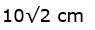cm

AO = OC = DO = OB =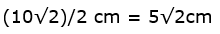cm

A charge of amount 1μC is placed at point O.

Force of repulsion between charges placed at corner A and centre O is equal in magnitude but opposite in direction relative to the force of repulsion between the charges placed at corner C and centre O. Hence, they will cancel each other. Similarly, force of attraction between charges placed at corner B and centre O is equal in magnitude but opposite in direction relative to the force of attraction between the charges placed at corner D and centre O. Hence, they will also cancel each other. Therefore, net force caused by the four charges placed at the corner of the square on 1 μC charge at centre O is zero.

Question7. (a) An electrostatic field line is a continuous curve. That is, a field line cannot have sudden breaks. Why not?

(b) Explain why two field lines never cross each other at any point?

Solution :
(a) An electrostatic field line is a continuous curve because a charge experiences a continuous force when traced in an electrostatic field. The field line cannot have sudden breaks because the charge moves continuously and does not jump from one point to the other.

(b) If two field lines cross each other at a point, then electric field intensity will show two directions at that point. This is not possible. Hence, two field lines never cross each other.

Question8. Two point charges qA = 3 μC and qB = −3 μC are located 20 cm apart in vacuum.

(a) What is the electric field at the midpoint O of the line AB joining the two charges?

(b) If a negative test charge of magnitude 1.5 × 10−9 C is placed at this point, what is the force experienced by the test charge?

Solution :
(a) The situation is represented in the given figure. O is the mid-point of line AB.

Distance between the two charges, AB = 20 cm

∴AO = OB = 10 cm

Net electric field at point O = E

Electric field at point O caused by +3μC charge,

E1 =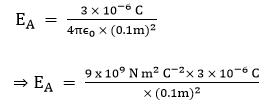along OB

Where,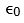= Permittivity of free space

Magnitude of electric field at point O caused by −3μC charge,

E2 =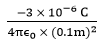=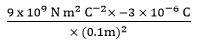along OB

= 5.4 × 106 N/C along OB

Therefore, the electric field at mid-point O is 5.4 × 106 N C−1 along OB.

(b) A test charge of amount 1.5 × 10−9 C is placed at mid-point O.

q = 1.5 × 10−9 C

Force experienced by the test charge = F

∴F = qE

= 1.5 × 10−9 × 5.4 × 106

= 8.1 × 10−3 N

The force is directed along line OA. This is because the negative test charge is repelled by the charge placed at point B but attracted towards point A.

Therefore, the force experienced by the test charge is 8.1 × 10−3 N along OA.

Question9. A system has two charges qA = 2.5 × 10−7 C and qB = −2.5 × 10−7 C located at points A: (0, 0, − 15 cm) and B: (0, 0, + 15 cm), respectively. What are the total charge and electric dipole moment of the system?

Solution :
Both the charges can be located in a coordinate frame of reference as shown in the given figure.

At A, amount of charge, qA = 2.5 × 10−7C

At B, amount of charge, qB = −2.5 × 10−7 C

Total charge of the system,

q = qA + qB

= 2.5 × 10−7 C − 2.5 × 10−7 C

= 0

Distance between two charges at points A and B,

d = 15 + 15 = 30 cm = 0.3 m

Electric dipole moment of the system is given by,

p = qA × d = qB × d

= 2.5 × 10−7 × 0.3

= 7.5 × 10−8 C m along positive z-axis

Therefore, the electric dipole moment of the system is 7.5 × 10−8 C m along positive z−axis.

Question10. An electric dipole with dipole moment 4 × 10−9 C m is aligned at 30° with the direction of a uniform electric field of magnitude 5 × 104 N C−1. Calculate the magnitude of the torque acting on the dipole.

Solution :
Electric dipole moment, p = 4 × 10−9 C m

Angle made by p with a uniform electric field, θ = 30°

Electric field, E = 5 × 104 N C−1

Torque acting on the dipole is given by the relation,

τ = pE sinθ

Therefore, the magnitude of the torque acting on the dipole is 10−4 N m.

Question11. A polythene piece rubbed with wool is found to have a negative charge of 3 × 10−7 C.

(a) Estimate the number of electrons transferred (from which to which?)

(b) Is there a transfer of mass from wool to polythene?

Solution :
(a) When polythene is rubbed against wool, a number of electrons get transferred from wool to polythene. Hence, wool becomes positively charged and polythene becomes negatively charged.

Amount of charge on the polythene piece, q = −3 × 10−7 C

Amount of charge on an electron, e = −1.6 × 10−19 C

Number of electrons transferred from wool to polythene = n

n can be calculated using the relation,

q = ne

= 1.87 × 1012

Therefore, the number of electrons transferred from wool to polythene is 1.87 × 1012.

(b) Yes.

There is a transfer of mass taking place. This is because an electron has mass,

me = 9.1 × 10−3 kg

Total mass transferred to polythene from wool,

m = me × n

= 9.1 × 10−31 × 1.85 × 1012

= 1.706 × 10−18 kg

Hence, a negligible amount of mass is transferred from wool to polythene.

Question12. (a) Two insulated charged copper spheres A and B have their centers separated by a distance of 50 cm. What is the mutual force of electrostatic repulsion if the charge on each is 6.5 × 10−7 C? The radii of A and B are negligible compared to the distance of separation.

(b) What is the force of repulsion if each sphere is charged double the above amount, and the distance between them is halved?

Solution :
(a) Charge on sphere A, qA = Charge on sphere B, qB = 6.5 × 10−7 C

Distance between the spheres, r = 50 cm = 0.5 m

Force of repulsion between the two spheres,

Where,

0 = Free space permittivity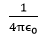= 9 × 109 N m2 C−2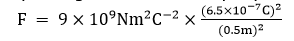= 1.52 × 10−2 N

Therefore, the force between the two spheres is 1.52 × 10−2 N.

(b) After doubling the charge, charge on sphere A, qA = Charge on sphere B, qB = 2 × 6.5 × 10−7 C = 1.3 × 10−6 C

The distance between the spheres is halved.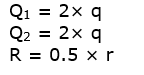Force of repulsion between the two spheres,

= 16 × 1.52 × 10−2

= 0.243 N

Therefore, the force between the two spheres is 0.243 N.

Question13. Suppose the spheres A and B in Exercise 1.12 have identical sizes. A third sphere of the same size but uncharged is brought in contact with the first, then brought in contact with the second, and finally removed from both. What is the new force of repulsion between A and B?

Solution :

Note: If a conducting uncharged material is brought in contact with a charged surface then the charges are shared uniformly between the two bodies.

Given: Charge on sphere 1, q1 = 6.5× 10-7 C

Charge on sphere 2, q2 = 6.5× 10-7 C

Charge on sphere 3, q3 = 0

Step 1: The uncharged sphere is brought in contact with sphere 1. Since sphere 1 has charge ‘q’, it gets distributed among sphere 1 and sphere 3.

Now, charge on sphere 1 = q/2

Charge on sphere 2 = q/2

At this point the sphere 3 which was initially uncharged has a charge “q/2”.

Step 2: Now sphere 3 is brought in contact with sphere 2 due to which 1/4 × q will flow from sphere 2 to sphere 3. Now sphere 2 and sphere 3 have “3/4 × q” charge.

Now, q1 = ½ × 6.5 × 10-7 C

⇒ 3.25× 10-7 C

q2 = ¾ × 6.5× 10-7 C

⇒ 4.87 × 10-7 C

q3 = ¾ × 6.5× 10-7 C

⇒ 4.87 × 10-7 C

Question14. Figure 1.33 shows tracks of three charged particles in a uniform electrostatic field. Give the signs of the three charges. Which particle has the highest charge to mass ratio?

Solution :

Opposite charges attract each other and same charges repel each other. It can be observed that particles 1 and 2 both move towards the positively charged plate and repel away from the negatively charged plate. Hence, these two particles are negatively charged. It can also be observed that particle 3 moves towards the negatively charged plate and repels away from the positively charged plate. Hence, particle 3 is positively charged.

The charge to mass ratio (emf) is directly proportional to the displacement or amount of deflection for a given velocity. Since the deflection of particle 3 is the maximum, it has the highest charge to mass ratio.

Question15. Consider a uniform electric field E = 3 × 103 îN/C. (a) What is the flux of this field through a square of 10 cm on a side whose plane is parallel to the yz plane? (b) What is the flux through the same square if the normal to its plane makes a 60° angle with the x-axis?

Solution :Given:

Electric field E = 3× 103  N/C

Side of square, s = 10 cm

a)  Flux of field through square whose plane is parallel to yz plane.

We understand that the normal to the plane is parallel to the direction of field.

So, θ = 0°

Ø = E . A

Ø = E × A× cos(θ)            …(1)

Where, E = Electric field

A = Area through which we have to calculate flux

θ = Angle between normal to surface and the Electric field

A = s2

A = .01 m2

Plugging values, of E, A and θ in equation (1)

Ø = 3× 103 NC-1× 0.01m2× cos0°

Ø = 30 Nm2C-1

b)  If normal to its (square’s) plane makes 60° with the X axis.

θ = 60°

Ø = E × A× cos(θ)

Ø = 3× 103 NC-1× 0.01m2 × cos60°

Ø = 15 Nm2C-1

Question16. What is the net flux of the uniform electric field of Exercise 1.15 through a cube of side 20 cm oriented so that its faces are parallel to the coordinate planes?

Solution : All the faces of a cube are parallel to the coordinate axes. Therefore, the number of field lines entering the cube is equal to the number of field lines piercing out of the cube. As a result, net flux through the cube is zero.

Question17. Careful measurement of the electric field at the surface of a black box indicates that the net outward flux through the surface of the box is 8.0 × 103 N m2/C. (a) What is the net charge inside the box? (b) If the net outward flux through the surface of the box were zero, could you conclude that there were no charges inside the box? Why or Why not?

Solution :Given:

a)           Ø = 8.0× 103 Nm2C-1

Let net charge inside the box = q

We know that,

Flux, Ø = q/ε0                ..(1)

Where, q = net charged enclosed

ε0 = permittivity of free space

ε0 = 8.85× 10-12 N-1 m-2C2

Plugging values of Ø and εin equation (1) we get,

q = Ø × ε0

⇒ q = 8.0 × 103 Nm2C-1× 8.85× 10-12N-1C2m-2

⇒ q = 7.08 × 10-8 C

Hence, the net charge inside the box is 0.07 µC.

(b) No, we cannot conclude that the body doesn’t have any charge. The flux is due to the Net charge of the body. There may still be equal amount of positive and negative charges. So, it is not necessary that if flux is zero then there will be no charges.

Question18. A point charge +10 μC is a distance 5 cm directly above the centre of a square of side 10 cm, as shown in Fig. 1.34. What is the magnitude of the electric flux through the square? (Hint: Think of the square as one face of a cube with edge 10 cm.)

Solution :Given:

q = + 10

s = 10 cm

Assume the charge to be enclosed by a cube, where the square is one of its sides.

Now, let us find the total flux through the imaginary cube.

We know that,

Flux, Ø = q/ε            …(1)

Where, q = net charged enclosed

ε0 = permittivity of free space

ε0 = 8.85× 10-12 N-1 m-2C2

Now plugging the values of q and εin equation (2)

⇒ Ø = 11.28 × 105 Nm-2C-1

We understand that flux through all the faces of cube will be equal;

Let flux through the square = Øa

Hence,

Øa = Ø/6

Explanation: The net flux will be distributed equally among all 6 faces of the cube. Hence, the square will have one sixth of the total flux.

Øa = 1.88 Nm-2C-1

Question19. A point charge of 2.0 μC is at the centre of a cubic Gaussian surface 9.0 cm on edge. What is the net electric flux through the surface?

Solution :Given:

Total charge inside the cube, q = 2.0 µC

Edge length of Cube, a = 9.0 cm

Question20. A point charge causes an electric flux of −1.0 × 103 Nm2/C to pass through a spherical Gaussian surface of 10.0 cm radius centered on the charge. (a) If the radius of the Gaussian surface were doubled, how much flux would pass through the surface? (b) What is the value of the point charge?

Solution :Given:

Ø = -1.0× 103 Nm2C-1

r­1 = 10.0 cm.

a)  Flux if the radius of the Gaussian surface is doubled.

If the radius is doubled then the flux would remain same i.e. -1.0× 103 Nm2C-1.

The geometry of the Gaussian surface doesn’t affect the total flux through it. The net charge enclosed by Gaussian surface determines the net flux.

b)  Let value of point charge enclosed by Gaussian surface = q

We know that,

Flux, Ø = q/ε0           …(1)

Where, q = net charged enclosed

ε0 = permittivity of free space

ε0 = 8.85× 10-12 N-1 m-2C2

Now plugging, the values Ø and εin equation (1).

q = Ø× ε0

⇒ q = -1.0× 103Nm2C-1 × 8.85× 10-12N-1m-2C2

⇒ q = -8.85 × 10-9 C

The charge enclosed by the surface is -8.85 × 10-9 C.

Question21. A conducting sphere of radius 10 cm has an unknown charge. If the electric field 20 cm from the centre of the sphere is 1.5 × 10N/C and points radially inward, what is the net charge on the sphere?

Solution :Given:

Radius of charged sphere, r = 10 cm

Electric field, 20 cm away from centre of sphere, E = 1.5× 10NC-1

We know that, electric field intensity at a point P, located at a distance R, due to net charge q is given by,

…(1)

Now plugging the values of q and R in equation (1)

q = 4× π× ε0× R2× E

⇒ q = 4× 3.14× 8.85× 10-12N-1m-2C-2× (0.2m)2 × 1.5× 103C

⇒ q = 6.67× 10-9C

The net charge on the sphere is -6.67× 10-9C. Since the electric field points radially inwards, we can infer that charges on sphere are negative.

Question22. A uniformly charged conducting sphere of 2.4 m diameter has a surface charge density of 80.0 μC/m2. (a) Find the charge on the sphere. (b) What is the total electric flux leaving the surface of the sphere?

Solution :Given: radius of sphere, r = 1.2 m

Surface charge density, σ = 80.0 μC/m2

a)  Let charge on sphere = q

We understand that,

Total charge, Q = Surface charge density× surface area.

Surface area of sphere, S = 4πr2

S = 18.08 m2

Q = 80× 10-6Cm-2× 18.08m-2

⇒ Q = 1.447 × 10-3C

b)  Let total electric flux leaving the surface of sphere =

We know that,

Flux, Ø = q/ε0           …(1)

Where, Q = net charged.

ε0 = permittivity of free space

ε0 = 8.85× 10-12 N-1 m-2C2

By, plugging the values of q and εin equation (1), we get,(b)

Question23. An infinite line charge produces a field of 9 × 104 N/C at a distance of 2 cm. Calculate the linear charge density.

Solution :Given:

E = 9× 104 NC-1

d = 2 cm

Let the linear charge density = λ Coulomb/metre

Where, λ = linear charge density.

ε0 = permittivity of free space

ε0 = 8.85× 10-12 N-1 m-2C2

d = distance

From equation (1) we have,

⇒ λ = E× 2× π× ε0× d

⇒ λ = 9× 104 NC-1× 2× π×8.85× 10-12 N-1 m-2C2× 0.02m

⇒ λ = 10 µ Cm-1

The linear charge density is 10 µ Cm-1.

Question24. Two large, thin metal plates are parallel and close to each other. On their inner faces, the plates have surface charge densities of opposite signs and of magnitude 17.0 × 10−22 C/m2. What is E: (a) in the outer region of the first plate, (b) in the outer region of the second plate, and (c) between the plates?

Solution :Given:

Surface charge density on plate A, σA = -17.0 × 10-22 Cm-2

Surface charge density on plate B, σB = 17.0 × 10-22 Cm-2

The arrangement of plates are as shonw:

Let electric field in region 1 = E1

Region 2 = E2

Region 3 = E3

The electric field in region I and region III is zero because no charge is present in these regions.

E1 = 0

E2 = 0

We know that,

E3 = σ/ε0   …(1)

Where, σ Surface charge density

ε0 = permittivity of free space

ε0 = 8.85× 10-12 N-1 m-2C2

Now, plugging the Values in equation (1).

⇒ E3 = 1.92 × 10-10 NC-1

The electric field in the region enclosed by the plates is found to be 1.92 × 10-10 NC-1.

The electric field in region III is 1.92 × 10-10 NC-1.

Question25. An oil drop of 12 excess electrons is held stationary under a constant electric field of 2.55 × 104 N C−1 in Millikan’s oil drop experiment. The density of the oil is 1.26 g cm−3. Estimate the radius of the drop. (g = 9.81 m s−2; e = 1.60 × 10−19 C).

Solution :If the oil drop is stationary, the net force on it must be Zero or the resultant of all the forces on oil drop is zero. There are two forces acting on the oil drop, its weight or force due to Earth’s gravity which is pulling it vertically downwards, and Electrostatic force which is acting in vertically upward direction.Both forces should be equal in magnitude and opposite direction so that they cancel each other.

The arrangement is shown in the figure

Note: Electric Field is in vertically downward direction, because force on an negatively charged body in opposite direction of the field, so force on the drop is in vertically upward direction, which balances weight of the drop acting in vertically downward direction.

There are nine excess electrons which make the drop negatively charged because the electron is negatively charged, the net magnitude charge on a body is given by

q = n × E

n is the excess of electrons or protons on the body,

the charge of an electron is denoted by e

e = 1.60 × 10–19 C

here since there are twelve excess electrons so

n = 12

i.e. q = 12 × 1.60 × 10–19 C

= 1.92 × 10-18 C

Now the magnitude electrostatic force on a charged particle held in an electric field is given by

F = q × E

where, F force is acting on a particle having charge q held in an electric field of magnitude E

here, charge on the oil drop is

q = 1.92 × 10-18 C

magnitude of Electric field is

E = 2.55 × 104 NC–1

So Electrostatic force on the oil drop is

F = 1.92 × 10-18 C × 2.55 × 104 NC–1

= 4.896 ×10-14 N

This force is acting in vertically upward direction, so Weight should have same magnitude of Force and is acting in vertically downward direction.

Let us assume oil drop to be Spherical in shape, so the volume of drop will be

Both the forces should be equal in magnitude so equating them

i.e. putting F = W

4.896 ×10-14 N = (4/3 πr3) ×1.26 × 10Kgm-3 × 9.81ms-2

we get ,

Question26. Which among the curves shown in Fig. 1.35 cannot possibly represent electrostatic field lines?

(a)

(b)

(c)

(d)

(e)

Solution :

(a) The field lines showed in (a) do not represent electrostatic field lines because field lines must be normal to the surface of the conductor.

(b) The field lines showed in (b) do not represent electrostatic field lines because the field lines cannot emerge from a negative charge and cannot terminate at a positive charge.

(c) The field lines showed in (c) represent electrostatic field lines. This is because the field lines emerge from the positive charges and repel each other.

(d) The field lines showed in (d) do not represent electrostatic field lines because the field lines should not intersect each other.

(e) The field lines showed in (e) do not represent electrostatic field lines because closed loops are not formed in the area between the field lines.

Question27. In a certain region of space, electric field is along the z-direction throughout. The magnitude of electric field is, however, not constant but increases uniformly along the positive z-direction, at the rate of 105 NC−1 per metre. What are the force and torque experienced by a system having a total dipole moment equal to 10−7 Cm in the negative z-direction?

Solution :A dipole is a system consisting of two charges equal in magnitude and opposite in nature i.e. a positive charge + q and a negative charge –q separated by some distance d

Electric dipole moment of a dipole is given by,

P = q × d

Where, ‘q’ is magnitude of either of charge (in column)

And ‘d’ is separation between the pair of charges (in metres)

Dipole moment is a vector quantity and its direction is from negative charge to positive charge

We are given dipole moment of the system,

P = 10-7 Cm , along negative Z axis

Here Electric Field is varying at the rate of 105 NC–1 per metre in positive Z direction

i.e. dE/dl = 105 NC–1m-1 along Z direction

so the electric field at any point on z axis at distance of a metres

Force on a charged particle in an electric field is given by

F = q × E

Where q is the magnitude of charge and E is the magnitude of Electric Field and the force is same as the direction of electric field in case of a positively charged particle and opposite to the direction of field in case of negatively charged particle

so Force on the + q charge located at a distance l from origin will be

So whatever be the magnitude of P and E putting  in the equation  = PESin

We get the torque τ = 0 Nm

So the torque of the system is zero

Question28. (a) A conductor A with a cavity as shown in Fig. 1.36(a) is given a charge Q. Show that the entire charge must appear on the outer surface of the conductor. (b) Another conductor B with charge q is inserted into the cavity keeping B insulated from A. Show that the total charge on the outside surface of A is Q + q [Fig. 1.36(b)]. (c) A sensitive instrument is to be shielded from the strong electrostatic fields in its environment. Suggest a possible way.

Solution :

In Electrostatic we deal with charges at rest so there is no current inside or on the surface of the conductor i.e. in the static situation, the electric field is zero everywhere inside the conductor. This is the basic property of a conductor. A conductor has free electrons. As long as electric field is not zero, the free charge carriers would experience force and drift and re distribute themselves in such a way so that net electric field inside a conductor is zero.

We will use the above stated property to solve the problems

(a)Here in this situation a conductor with a cavity having no charge inside is given a charge Q on outer surface, we have to show that all charge resides on the external surface only and there is no charge on the inner surface.

From Gauss Theorem we know, net electric flux through an Gaussian surface enclosing a charge is equal to net charge enclosed by surface divided by permittivity of free space

we know that net electric field everywhere inside a conductor is zero

so the electric flux through this Gaussian surface would also be zero, this means no charge is enclosed by the Gaussian surface, since cavity does not contain any charge so no charge should be induced on the cavity’s metal surface as well so whole of the charge Q is distributed on the outer surface only.

(b) Again using Gauss Theorem we know,

If electric field at all the points on a Gaussian surface is zero then the net charge enclosed by the Gaussian surface is also zero, or there is no charge inside the Gaussian surface

Now if we consider a Gaussian surface enclosing the cavity inside the conductor as shown in the figure

we know that net electric field everywhere inside a conductor is zero

so the electric flux through this Gaussian surface would also be zero, this means no charge is enclosed by the Gaussian surface, but the cavity has a charge q , this means a charge –q must be induced on the inner metal surface because net charge enclosed by Gaussian surface must be zero (q + (-q) = 0) , but the metal surface has only been given charge Q so net charge of system must only be q + Q , i.e. a charge q is induced on outer surface of the conductor as which makes the total charge on the conductor equal to Q only Q + (-q) + q = Q

and total charge of the system as

Q + (-q) + q + q = Q + q

This arrangement is also in accordance with the law of conservation of charge

note: Only Q charge has been given on the conductor’s outer surface , the charge appearing (Q + q) on it is only due to induction due to charge inside the cavity.

(c) To shield the sensitive instrument from strong electrostatic fields in its environment. We need to enclose it inside a metal piece’s Cavity, this process is also known as electrostatic shielding.

we consider a metal Block with a cavity having no charge inside and Instrument inside it as shown in the figure

any cavity inside a metal surface the electric field is zero no matter in which environment it is placed. So in order to save the Instrument from External Electrostatic fiels we need to keep it inside a metal Cavity.

Question30. Obtain the formula for the electric field due to a long thin wire of uniform linear charge density λ without using Gauss’s law. [Hint: Use Coulomb’s law directly and evaluate the necessary integral.]

Solution :Let AB be a long thin wire of uniform linear charge density λ.Let us consider the electric field intensity due to AB at point P at a distance h from it as shown in the figure.

Question31. It is now believed that protons and neutrons (which constitute nuclei of ordinary matter) are themselves built out of more elementary units called quarks. A proton and a neutron consist of three quarks each. Two types of quarks, the so called ‘up’ quark (denoted by u) of charge (+2/3) e, and the ‘down’ quark (denoted by d) of charge (−1/3) e, together with electrons build up ordinary matter. (Quarks of other types have also been found which give rise to different unusual varieties of matter.) Suggest a possible quark composition of a proton and neutron.

Solution :

Question32. (a) Consider an arbitrary electrostatic field configuration. A small test charge is placed at a null point (i.e., where E = 0) of the configuration. Show that the equilibrium of the test charge is necessarily unstable.

(b) Verify this result for the simple configuration of two charges of the same magnitude and sign placed a certain distance apart.

Solution :
(a) Let the equilibrium of the test charge be stable. If a test charge is in equilibrium and displaced from its position in any direction, then it experiences a restoring force towards a null point, where the electric field is zero. All the field lines near the null point are directed inwards towards the null point. There is a net inward flux of electric field through a closed surface around the null point. According to Gauss’s law, the flux of electric field through a surface, which is not enclosing any charge, is zero. Hence, the equilibrium of the test charge can be stable.

(b) Two charges of same magnitude and same sign are placed at a certain distance. The mid-point of the joining line of the charges is the null point. When a test charged is displaced along the line, it experiences a restoring force. If it is displaced normal to the joining line, then the net force takes it away from the null point. Hence, the charge is unstable because stability of equilibrium requires restoring force in all directions.

Question33. A particle of mass m and charge (−q) enters the region between the two charged plates initially moving along x-axis with speed vx (like particle 1 in Fig. 1.33). The length of plate is L and an uniform electric field E is maintained between the plates. Show that the vertical deflection of the particle at the far edge of the plate is qEL2/ (2mVx2).

Compare this motion with motion of a projectile in gravitational field discussed in Section 4.10 of Class XI Textbook of Physics.

Solution :Given,

Mass of the particle = m

Charge of the particle = -q

Velocity of the particle = vx

Length of the plate = L

Electric field between the plates = E

From Newton’s second law of motion,

This is the vertical displacement of the particle at the far edge of the plate.

This motion is very similar to the motion of a projectile in a gravitational field. In a gravitational field, the force acting on the particle is mg and in the given case it is qE. The trajectory followed by the object will be similar in both the cases.

NOTE: A projectile is any object thrown into space by the exertion of a force. The path followed by a projectile is known as its trajectory.

Question34. Suppose that the particle in Exercise in 1.33 is an electron projected with velocity vx= 2.0 × 106 m s−1. If E between the plates separated by 0.5 cm is 9.1 × 102 N/C, where will the electron strike the upper plate? (| e | =1.6 × 10−19 C, m= 9.1 × 10−31 kg.)

Solution :Given,

Velocity of the electron, v= 2.0 × 106 m s–1

Separation of plates, l = 0.5cm = 0.5 × 10-2 m

Electric field between the plates, E = 9.1 × 10N C-1

Charge on the electron, q = |e| = 1.6 × 10-19 C

Mass of the electron, me = 9.1 × 10-31 kg

The vertical deflection of the particle (s) is given by

Get 30% off your first purchase!

X
error: Content is protected !!
Scroll to Top